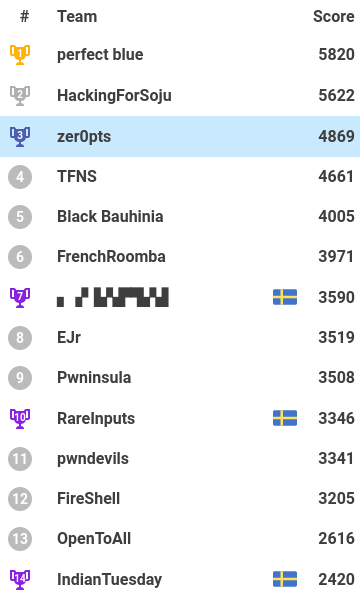# watevrCTF writeup

I played wateverCTF as a member of `zer0pts`. Luckily, my teammates are awesome. We reached 3rd place at the end of the competition!The scoreboard is pretty cool. And the infrastructure is so good. Moreover, there are very cool challenges. Thanks to all the admins!

## [Crypto] Swedish RSA

Also known as Polynomial RSA. This is just some abstract algebra for fun!

We got the following script and its result.

```flag = bytearray(raw_input())
flag = list(flag)
length = len(flag)
bits = 16

## Prime for Finite Field.
p = random_prime(2^bits-1, False, 2^(bits-1))

file_out.write("Prime: " + str(p) + "\n")

## Univariate Polynomial Ring in y over Finite Field of size p
R.<y> = PolynomialRing(GF(p))

## Analogous to the primes in Z
def gen_irreducable_poly(deg):
while True:
out = R.random_element(degree=deg)
if out.is_irreducible():
return out

## Polynomial "primes"
P = gen_irreducable_poly(ZZ.random_element(length, 2*length))
Q = gen_irreducable_poly(ZZ.random_element(length, 2*length))

## Public exponent key
e = 65537

## Modulus
N = P*Q
file_out.write("Modulus: " + str(N) + "\n")

## Univariate Quotient Polynomial Ring in x over Finite Field of size 659 with modulus N(x)
S.<x> = R.quotient(N)

## Encrypt
m = S(flag)
c = m^e

file_out.write("Ciphertext: " + str(c))
file_out.close()
```

Irreducible monic polynomials over the polynomial ring are the same as prime numbers over the integer. So RSA can be constructed on the polynomial ring. Thus same approach is available.

Fortunately (and I don't know why), the given N was factorized easily. Then just do it!

```p = 43753
PR.<y> = PolynomialRing(GF(p))

N = sage_eval(N.split(":"), locals=vars())

(P, _), ( Q, _ ) = N.factor()
n, m = P.degree(), Q.degree()
e = 65537
s = (p^n - 1)*(p^m - 1)
d = inverse_mod(e, s)

S.<x> = PR.quotient(N)
C = sage_eval(C.split(":"), locals=vars())

M = C^d
print("".join([chr(c) for c in M.list()]))
```

`watevr{RSA_from_ikea_is_fun_but_insecure#k20944uehdjfnjd335uro}`

## [Reversing] timeout

Stop, wait a minute!

We were given the ELF binary. When I executed it, it seemed to just exit. As I analyzed with IDA, it waits some seconds and calls `generate` with a `can_generate` global variable is 0x539 to yield the flag. But alarm prevents it.

So I used gdb to make `can_generate` 0x539 and call the `generate` function. I wrote it as a gdb script.

```# gdb -n -q -x solve.py ./timeout
import gdb
import re

gdb.execute("set pagination off")
variables = gdb.execute("info var", to_string=True)
for v in variables.split("\n"):
if not v.startswith("0x"):
continue
if name == "can_continue":
break
gdb.execute("break main")
gdb.execute("run")
gdb.execute("set {int}(" + addr + ") = 0x539")
gdb.execute("jump generate")
gdb.execute("quit")
```

`watevr{3ncrytion_is_overrated_youtube.com/watch?v=OPf0YbXqDm0}`

## [Reversing] Hacking For Vodka

We ran out of soju so you will have to hack for vodka now, smh my head! Anyways, good luck.

I got the ELF binary. It checked the flag is correct. But it branches depend on the result of `ptrace` system call. If I used a debugger or utilities like a `ltrace`, it derived to the wrong flag.

I used the angr's hook to return 0 from ptrace.

```import angr
import claripy

class PHook(angr.SimProcedure):
def run(self, args):
return claripy.BVV(0, 64)

p.hook_symbol("ptrace", PHook())

state = p.factory.entry_state()
simgr = p.factory.simulation_manager(state)
simgr.explore(find=0x400000 + 0xC4F)

try:
print(simgr.found.posix.dumps(0))
except:
`watevr{th4nk5_h4ck1ng_for_s0ju_hackingforsoju.team}`